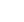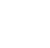# Engineering Mechanics

## Description

Are you interested in improving your mechanics or introducing yourself to the subject all together? Join our unique course, devised by the Ural Federal University. Through our innovative approach, you will receive the basic traditional material by engaging in practically-oriented tasks and learn the strictly theoretical mathematical analysis of basic concepts. You will be introduced to mathematical modelling of engineering designs, standard machines, and mechanisms using 2D and 3D diagrams. The course begins with statics, which is the science of forces. By the end of the course you will be able to: write down equilibrium conditions of structural elements and units of machines and mechanisms. perform the substitution of one system of forces that acts upon the structural elements and components of mechanisms with another equivalent one. create 2D and 3D diagrams of equilibrium in the standard engineering objects. choose appropriate mathematical models for calculating geometric parameters and force loads in the problems related to equilibrium of the engineering structures. apply combinations of mathematical operations according to the obtained mathematical models, when creating and solving equations describing equilibrium of the engineering structures. The weekly course includes video lectures on theoretical concepts, video demonstrations of the solutions of practical problems, tasks for students’ training, individual task, interim and final tests.

## Specific details

Category of Education Physical Science

## University

Ural Federal University

There are no comments posted here yetinfo@educom.net
 About Terms and ConditionsPrivacy PolicyRankingsAccreditations